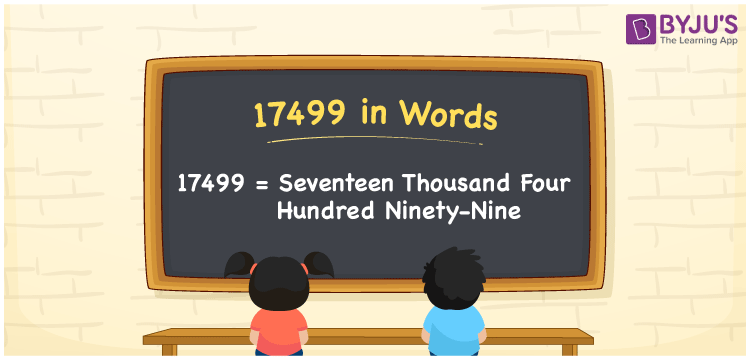# 17499 in Words

We can write 17499 in words as Seventeen thousand four hundred ninety-nine. Suppose you paid an EMI of Rs. 17499, then you can say, “I paid an EMI of Seventeen thousand four hundred ninety-nine rupees”. Also, we can say that 17499 is a cardinal number since it conveys a specific amount or quantity. Let’s learn how to convert the number 17499 into words here in this article.

 17499 in words Seventeen thousand four hundred ninety-nine Seventeen thousand four hundred ninety-nine in Numbers 17499

## 17499 in English Words

Generally, we write numbers in words using the English alphabet. Thus, we spell 17499 in English words as “Seventeen thousand four hundred ninety-nine”.## How to Write 17499 in Words?

As we can see, 17499 is a five-digit number. Thus, we need to create a five-column place value chart to convert 17499 into words. This can be done as given below.

 Ten thousand Thousands Hundreds Tens Ones 1 7 4 9 9

Here, ones = 9, tens = 9, hundreds = 4, thousands = 7, ten thousand = 1

By expanding these digits, we get;

1 × Ten thousand + 7 × Thousand + 4 × Hundred + 9 × Ten + 9 × One

= 1 × 10000 + 7 × 1000 + 4 × 100 + 9 × 10 + 9 × 1

= 10000 + 7000 + 400 + 90 + 9

= Ten Thousand + Seven thousand + Four hundred + Ninety + Nine

= Seventeen thousand + Four hundred ninety-nine

= Seventeen thousand four hundred ninety-nine

Hence, 17499 in words = Seventeen thousand four hundred ninety-nine.

17499 is a natural number that is the successor of 17498 and predecessor of 17500.

17499 in words – Seventeen thousand four hundred ninety-nine

Is 17499 an even number? – No

Is 17499 an odd number? – Yes

Is 17499 a prime number? – No

Is 17499 a composite number? – Yes

Is 17499 a perfect square number? – No

Is 17499 a perfect cube number? – No

## Frequently Asked Questions on 17499 in Words

Q1

### How do you write 17499 in words?

Using English words, we spell 17499 as Seventeen thousand four hundred ninety-nine.
Q2

### How to express Rs. 17499 in words on a cheque?

On a cheque, we can express Rs. 17499 in words as “Seventeen thousand four hundred ninety-nine rupees”.
Q3

### What is the number name of 17499?

The number name of 17499 is given by Seventeen thousand four hundred ninety-nine.